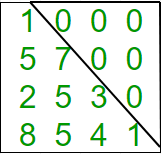# Program to check if matrix is lower triangular

Given a square matrix and the task is to check the matrix is in lower triangular form or not. A square matrix is called lower triangular if all the entries above the main diagonal are zero.Examples:

```Input : mat = {{1, 0, 0, 0},
{1, 4, 0, 0},
{4, 6, 2, 0},
{0, 4, 7, 6}};
Output : Matrix is in lower triangular form.

Input : mat = {{1, 0, 0, 0},
{4, 3, 0, 1},
{7, 9, 2, 0},
{8, 5, 3, 6}};
Output : Matrix is not in lower triangular form.
```

## C++

 `// Program to check lower ` `// triangular matrix. ` `#include ` `#define N 4 ` `using` `namespace` `std; ` ` `  `// Function to check matrix is in  ` `// lower triangular form or not. ` `bool` `isLowerTriangularMatrix(``int` `mat[N][N]) ` `{ ` `    ``for` `(``int` `i = 0; i < N; i++) ` `        ``for` `(``int` `j = i + 1; j < N; j++) ` `            ``if` `(mat[i][j] != 0) ` `                ``return` `false``; ` `    ``return` `true``; ` `} ` ` `  `// Driver function. ` `int` `main() ` `{ ` `    ``int` `mat[N][N] = { { 1, 0, 0, 0 }, ` `                      ``{ 1, 4, 0, 0 }, ` `                      ``{ 4, 6, 2, 0 }, ` `                      ``{ 0, 4, 7, 6 } }; ` ` `  `    ``// Function call ` `    ``if` `(isLowerTriangularMatrix(mat)) ` `        ``cout << ``"Yes"``; ` `    ``else` `        ``cout << ``"No"``; ` `    ``return` `0; ` `} `

## Java

 `// Java Program to check for  ` `// a lower triangular matrix. ` `import` `java.io.*; ` ` `  `class` `Lower_triangular ` `{ ` `    ``int` `N = ``4``; ` ` `  `    ``// Function to check matrix is  ` `    ``// in lower triangular form or not. ` `    ``boolean` `isLowerTriangularMatrix(``int` `mat[][]) ` `    ``{ ` `        ``for` `(``int` `i = ``0``; i < N; i++) ` `            ``for` `(``int` `j = i + ``1``; j < N; j++) ` `                ``if` `(mat[i][j] != ``0``) ` `                    ``return` `false``; ` ` `  `        ``return` `true``; ` `    ``} ` ` `  `    ``// Driver function. ` `    ``public` `static` `void` `main(String args[]) ` `    ``{ ` `        ``Lower_triangular ob = ``new` `Lower_triangular(); ` `        ``int` `mat[][] = { { ``1``, ``0``, ``0``, ``0` `}, ` `                        ``{ ``1``, ``4``, ``0``, ``0` `}, ` `                        ``{ ``4``, ``6``, ``2``, ``0` `}, ` `                        ``{ ``0``, ``4``, ``7``, ``6` `} }; ` `                         `  `        ``// Function call ` `        ``if` `(ob.isLowerTriangularMatrix(mat)) ` `            ``System.out.println(``"Yes"``); ` `        ``else` `            ``System.out.println(``"No"``); ` `    ``} ` `} ` ` `  `// This code is contributed by Anshika Goyal. `

## Python3

 `# Python3 Program to check  ` `# lower triangular matrix. ` ` `  `# Function to check matrix  ` `# is in lower triangular ` `def` `islowertriangular(M): ` `    ``for` `i ``in` `range``(``0``, ``len``(M)): ` `        ``for` `j ``in` `range``(i ``+` `1``, ``len``(M)): ` `            ``if``(M[i][j] !``=` `0``):  ` `                    ``return` `False` `    ``return` `True` `     `  `# Driver function. ` `M ``=` `[[``1``,``0``,``0``,``0``], ` `     ``[``1``,``4``,``0``,``0``], ` `     ``[``4``,``6``,``2``,``0``], ` `     ``[``0``,``4``,``7``,``6``]] ` ` `  `if` `islowertriangular(M): ` `    ``print` `(``"Yes"``) ` `else``: ` `    ``print` `(``"No"``) ` `     `  `# This code is contributed by Anurag Rawat `

## C#

 `// C# program to check for ` `// a lower triangular matrix. ` `using` `System; ` ` `  `class` `Lower_triangular  ` `{ ` `    ``int` `N = 4; ` ` `  `    ``// Function to check matrix is ` `    ``// in lower triangular form or not. ` `    ``bool` `isLowerTriangularMatrix(``int``[, ] mat) ` `    ``{ ` `        ``for` `(``int` `i = 0; i < N; i++) ` `            ``for` `(``int` `j = i + 1; j < N; j++) ` `                ``if` `(mat[i, j] != 0) ` `                    ``return` `false``; ` ` `  `        ``return` `true``; ` `    ``} ` ` `  `    ``// Driver function. ` `    ``public` `static` `void` `Main() ` `    ``{ ` `        ``Lower_triangular ob = ``new` `Lower_triangular(); ` `        ``int``[, ] mat = { { 1, 0, 0, 0 }, ` `                        ``{ 1, 4, 0, 0 }, ` `                        ``{ 4, 6, 2, 0 }, ` `                        ``{ 0, 4, 7, 6 } }; ` ` `  `        ``// Function call ` `        ``if` `(ob.isLowerTriangularMatrix(mat)) ` `            ``Console.WriteLine(``"Yes"``); ` `        ``else` `            ``Console.WriteLine(``"No"``); ` `    ``} ` `} ` ` `  `// This code is contributed by vt_m. `

## PHP

 ` `

Output:

```Yes
```

Attention reader! Don’t stop learning now. Get hold of all the important DSA concepts with the DSA Self Paced Course at a student-friendly price and become industry ready.

My Personal Notes arrow_drop_upCheck out this Author's contributed articles.

If you like GeeksforGeeks and would like to contribute, you can also write an article using contribute.geeksforgeeks.org or mail your article to contribute@geeksforgeeks.org. See your article appearing on the GeeksforGeeks main page and help other Geeks.

Please Improve this article if you find anything incorrect by clicking on the "Improve Article" button below.

Improved By : jit_t

Article Tags :
Practice Tags :

1

Please write to us at contribute@geeksforgeeks.org to report any issue with the above content.